# AP SSC Board Question Paper for Class 10th Science Paper I 2017 In PDF

## AP 10th Standard Science Paper I Exam Question Papers 2017 with Solutions – Free Download

The AP Board Class 10 Science Paper I 2017 Question Paper with solutions is given in the PDF format, below in this article. These AP SSC question paper solutions help the students to practise well for the board exams. The Class 10 exam question paper is the perfect resource that helps the students to ace the Science exams easily. With just a click, students can now download the PDF formats of the solved or the unsolved question paper, as they prefer. The clickable links listed below in this article, are thus considered as a very useful resource to prepare for the exams.

For students who are aspiring to gain high scores in Science, mastering all the complex concepts related to the subject thoroughly is the best method to adapt. Students can easily ace the AP Board Class 10 Science Part I exam if they know well the complex formulas and equations of the subject. Students are advised to revise the entire subject before the exam and solve the previous papers of AP Board Class 10 Science Part I to get an overview of the exam pattern. This will make them more familiar with the various question types that are likely to be asked in the exams. Students will also be able to gauge the difficulty level of the exams from these question paper solutions.

### AP Board Class 10 Science Part I 2017 Question Paper with Solutions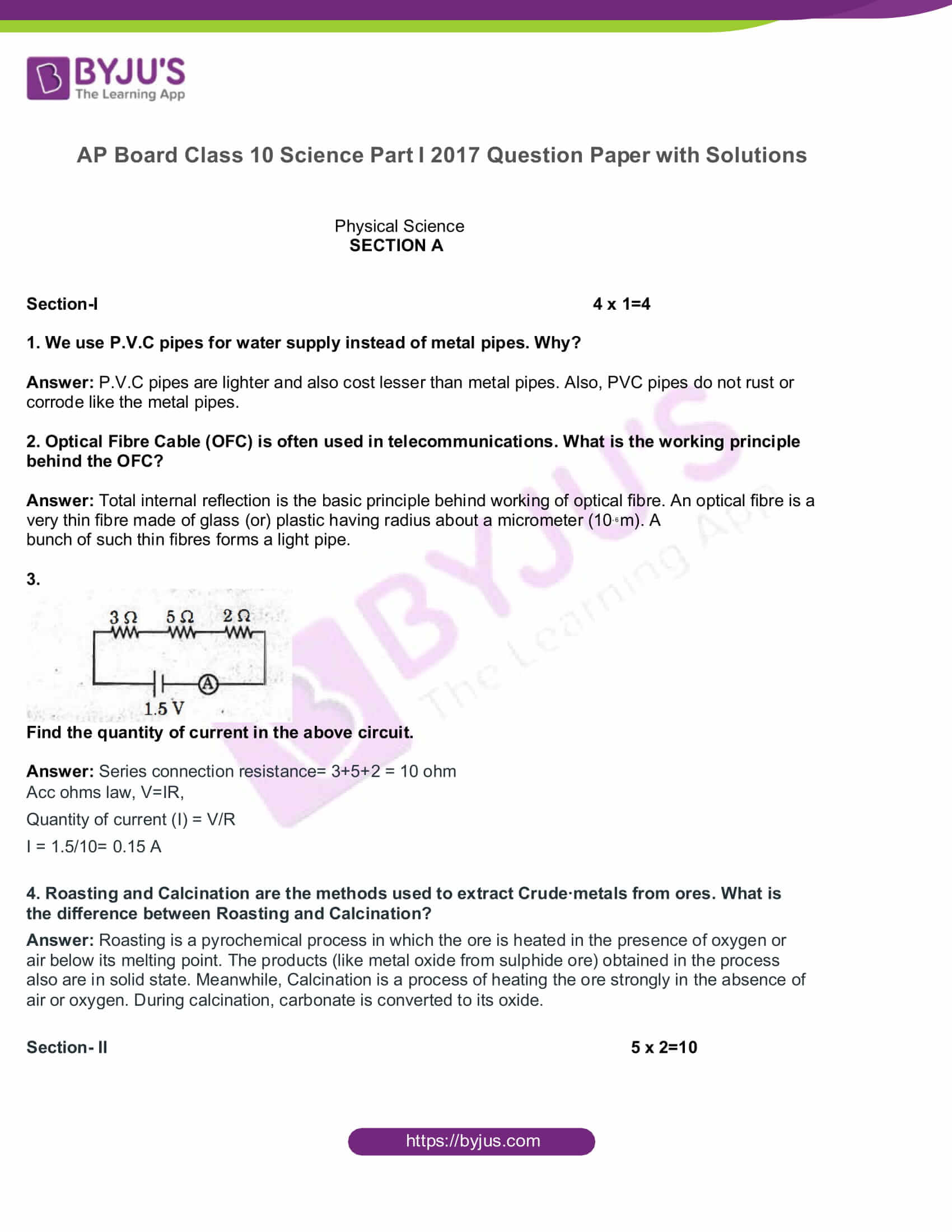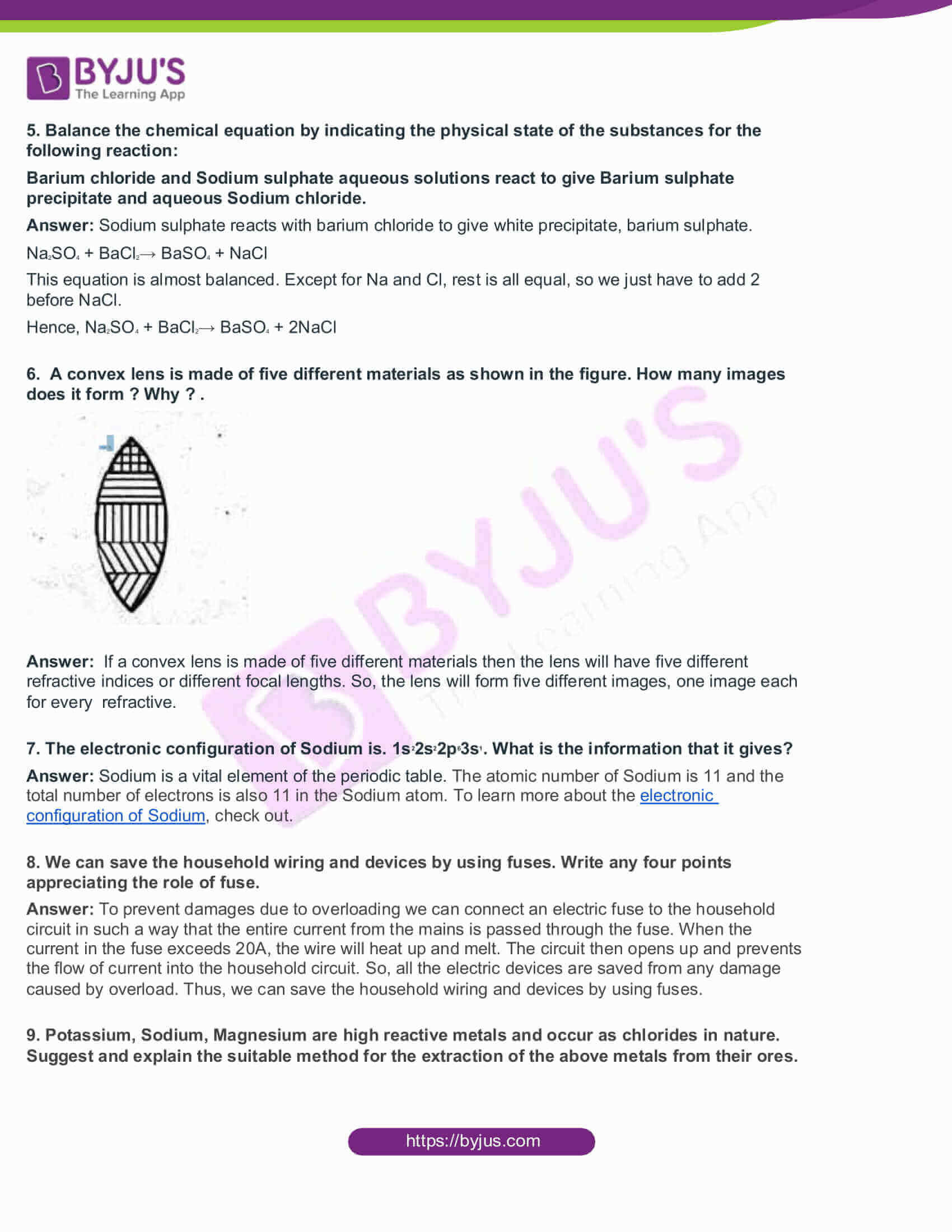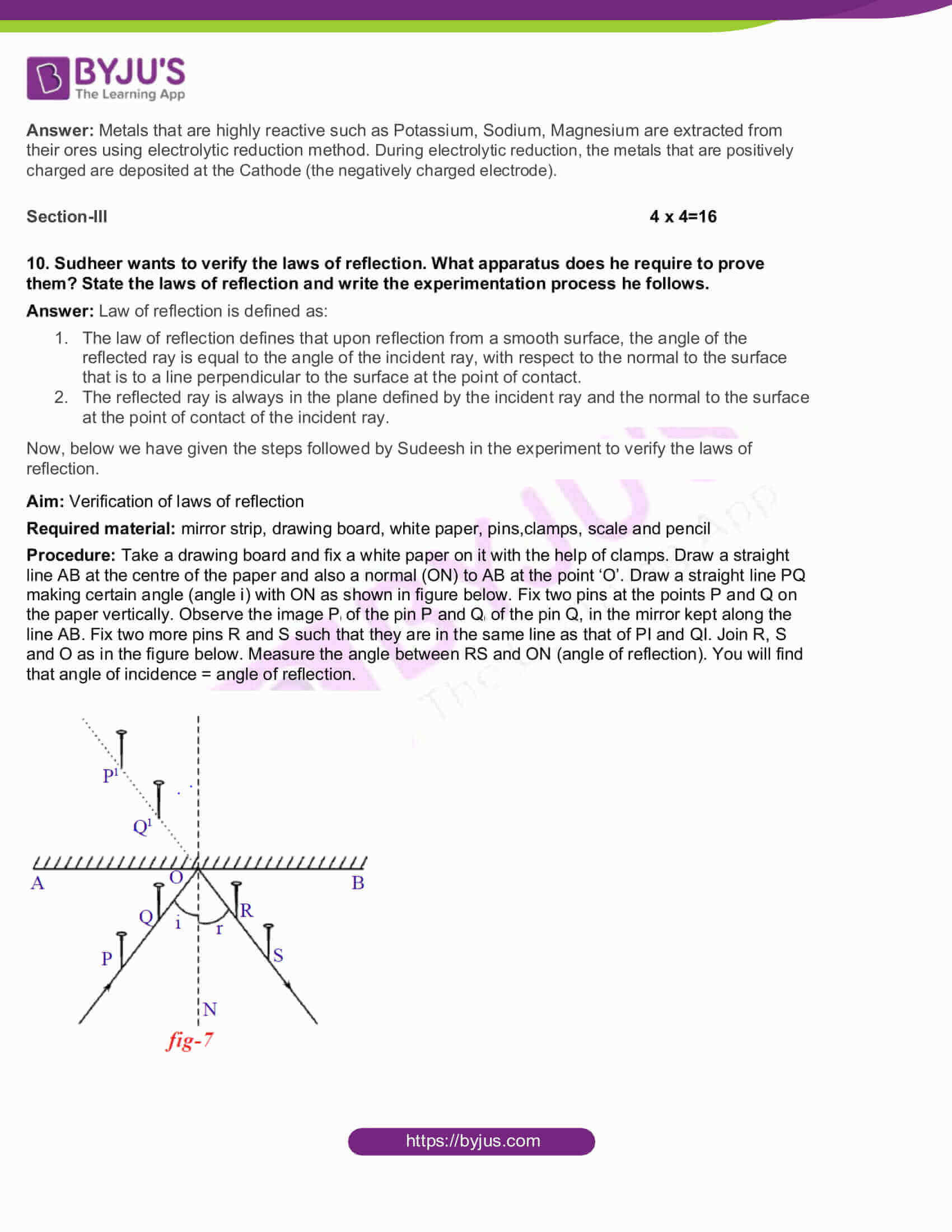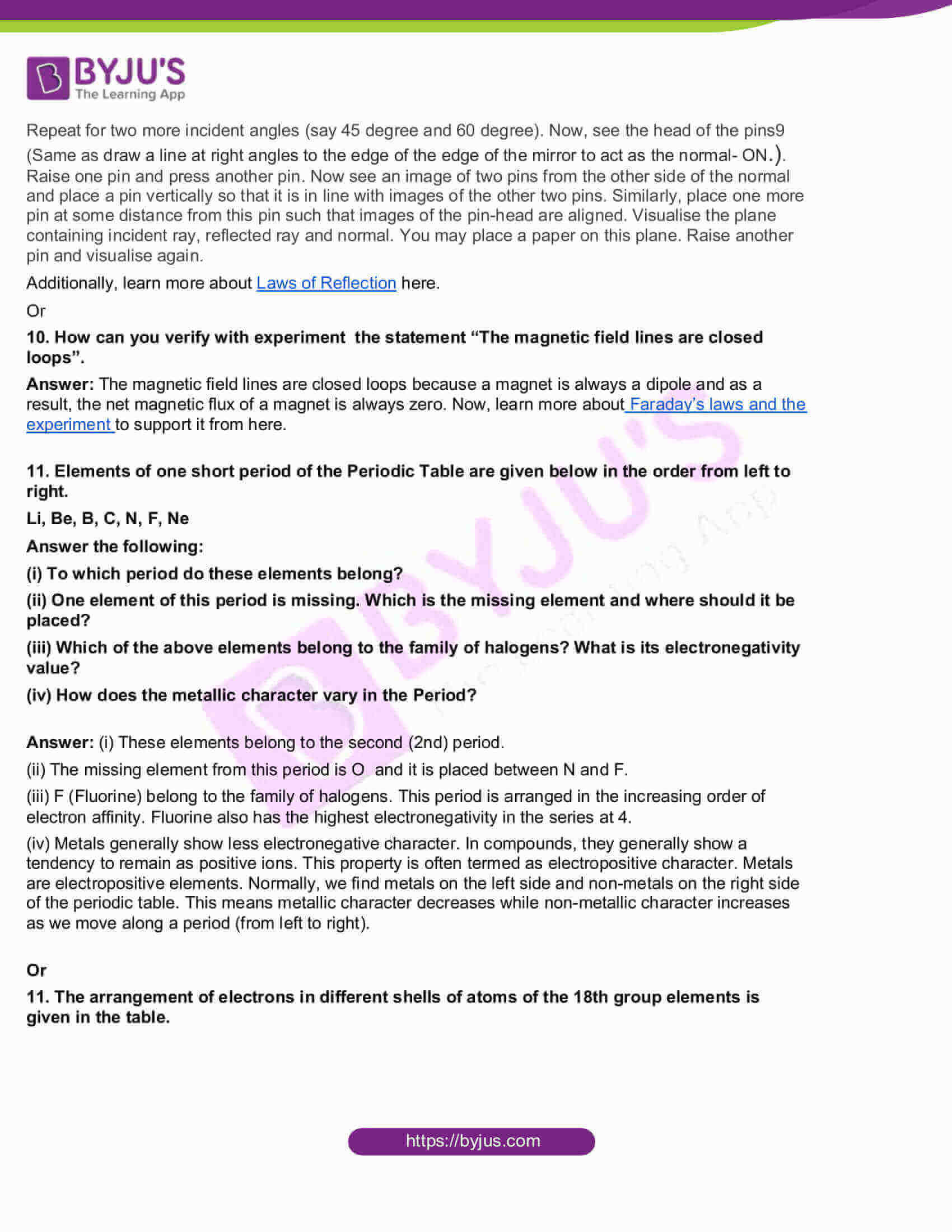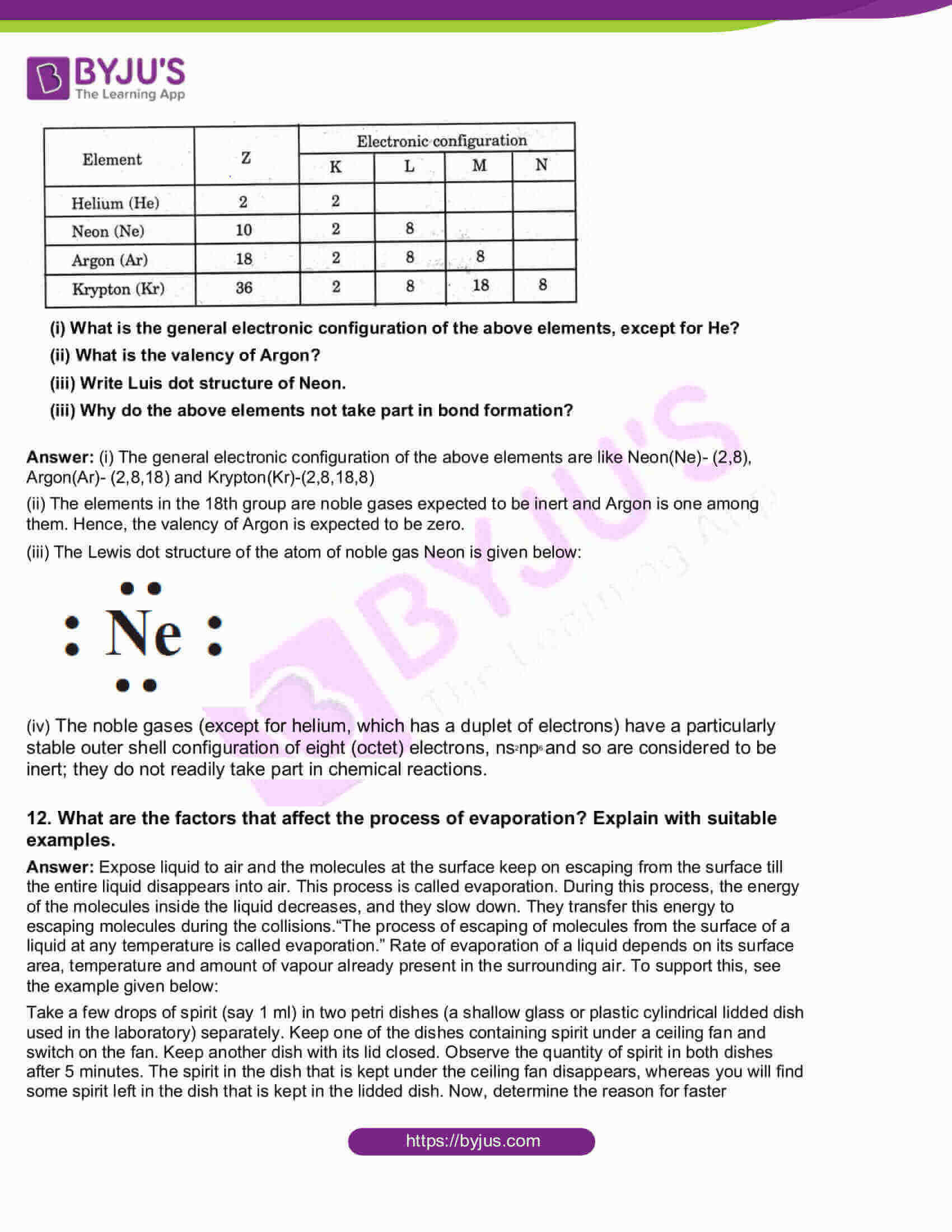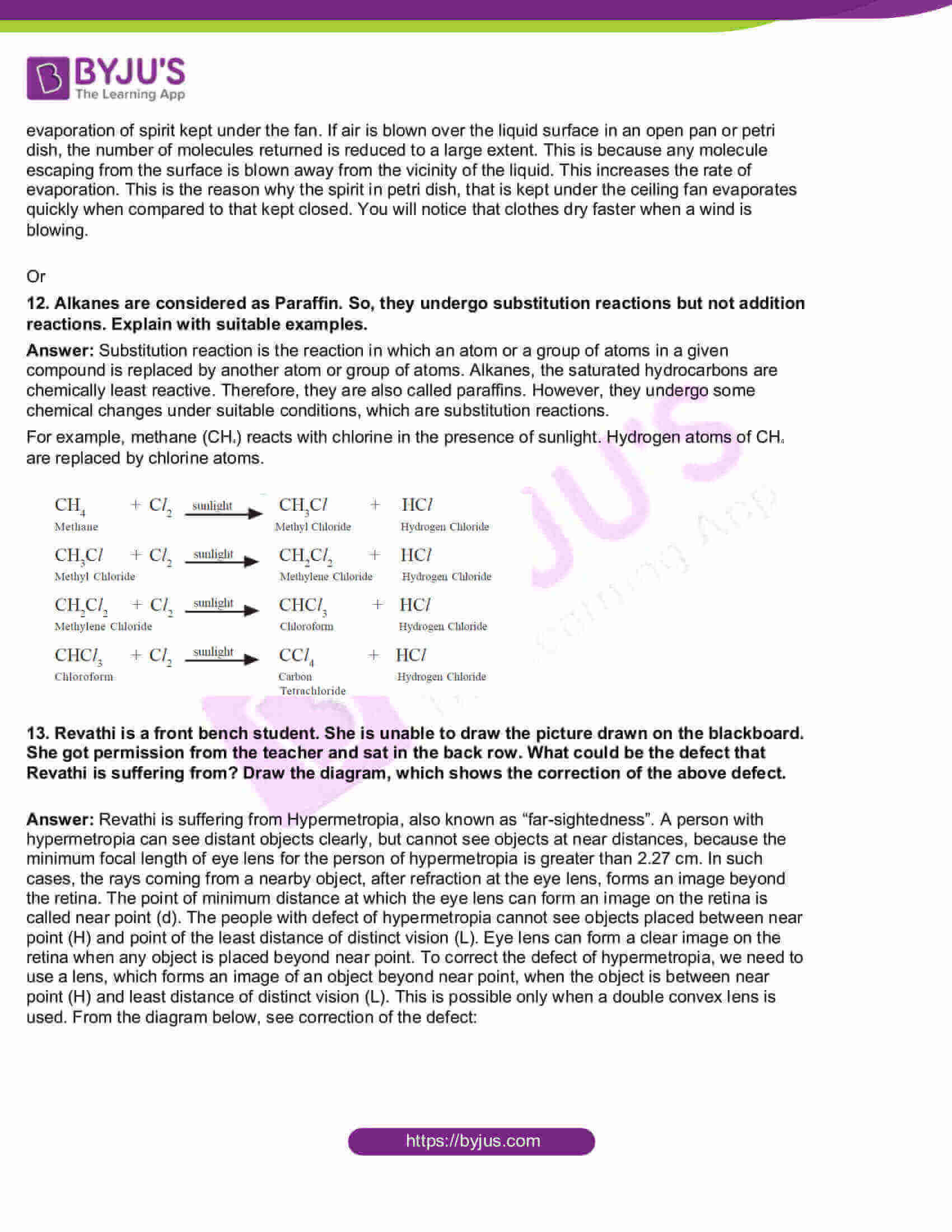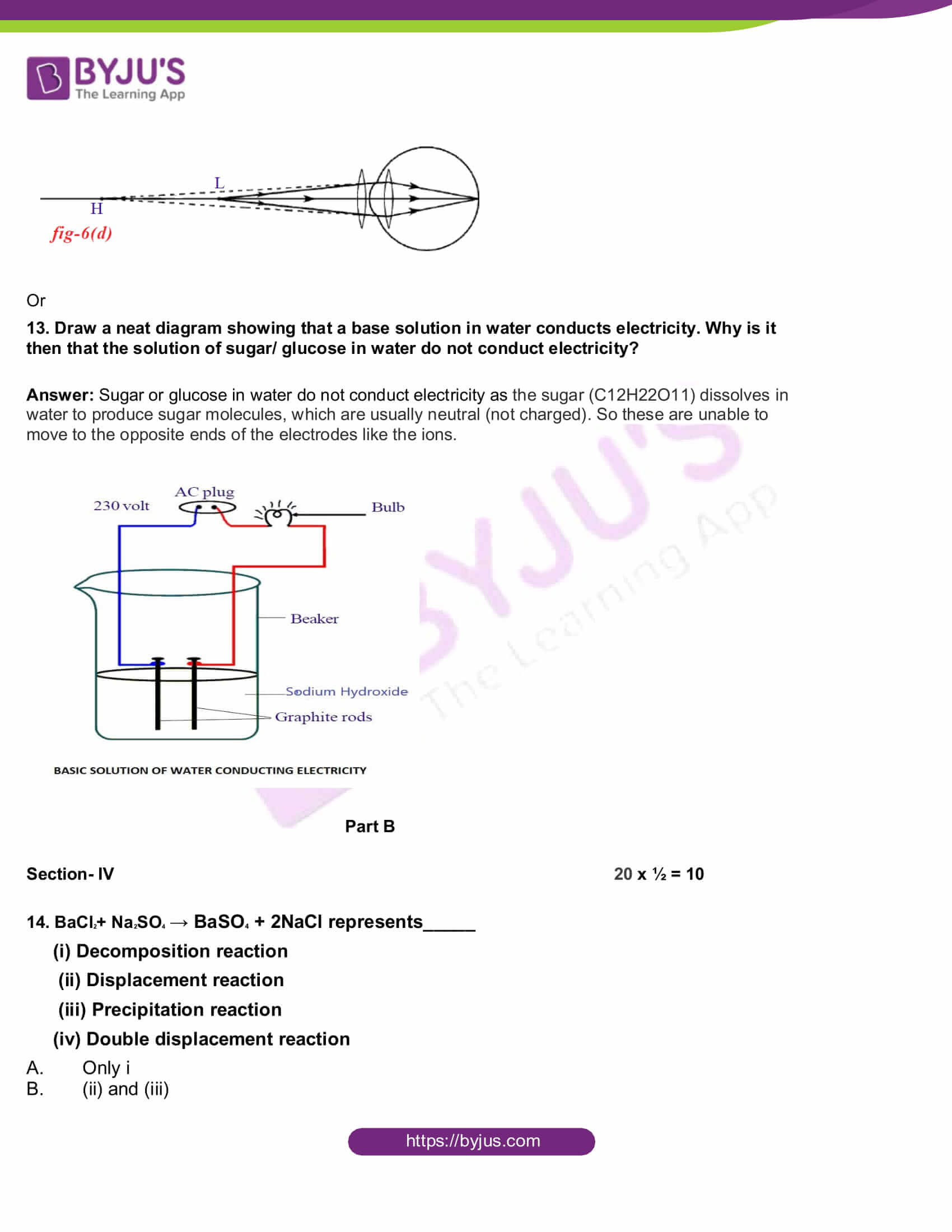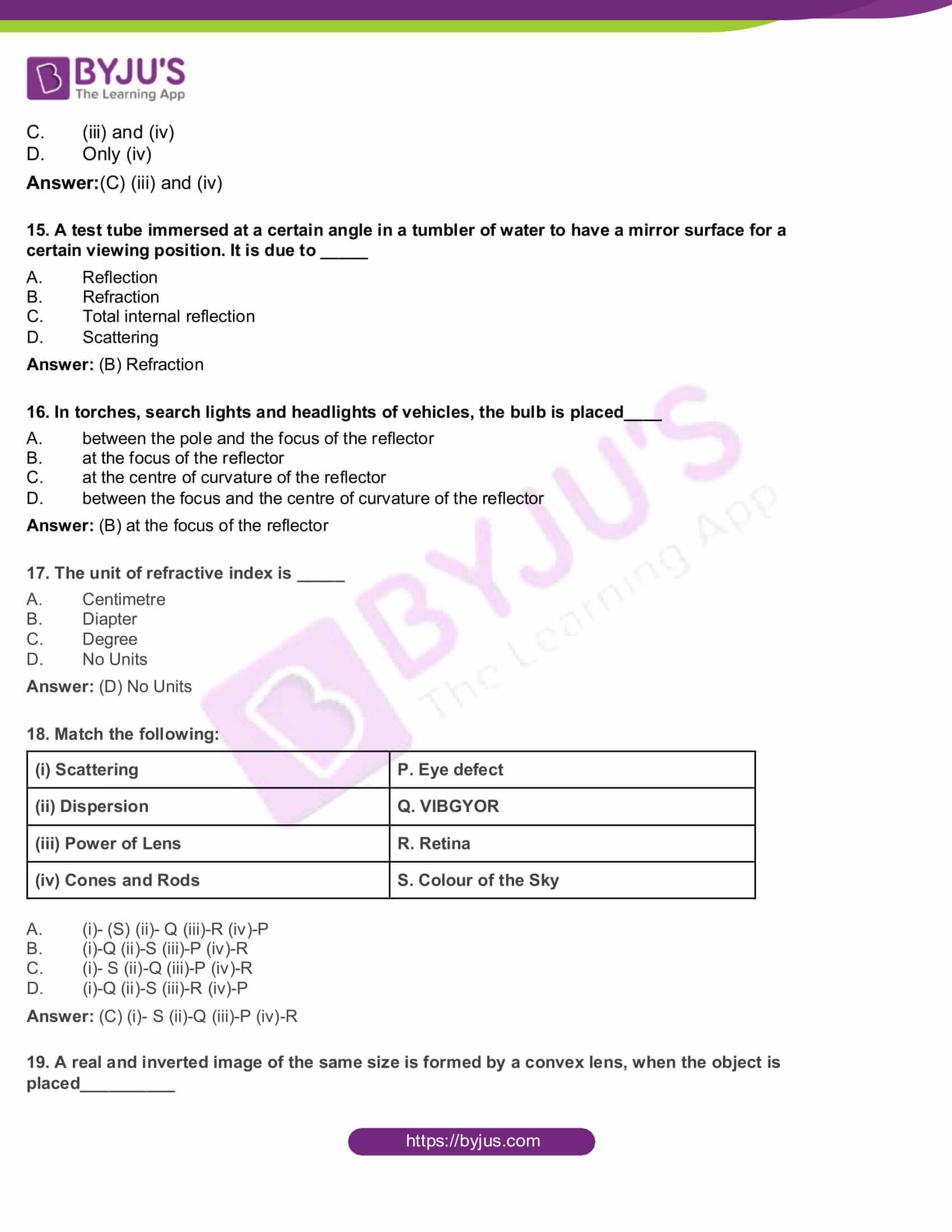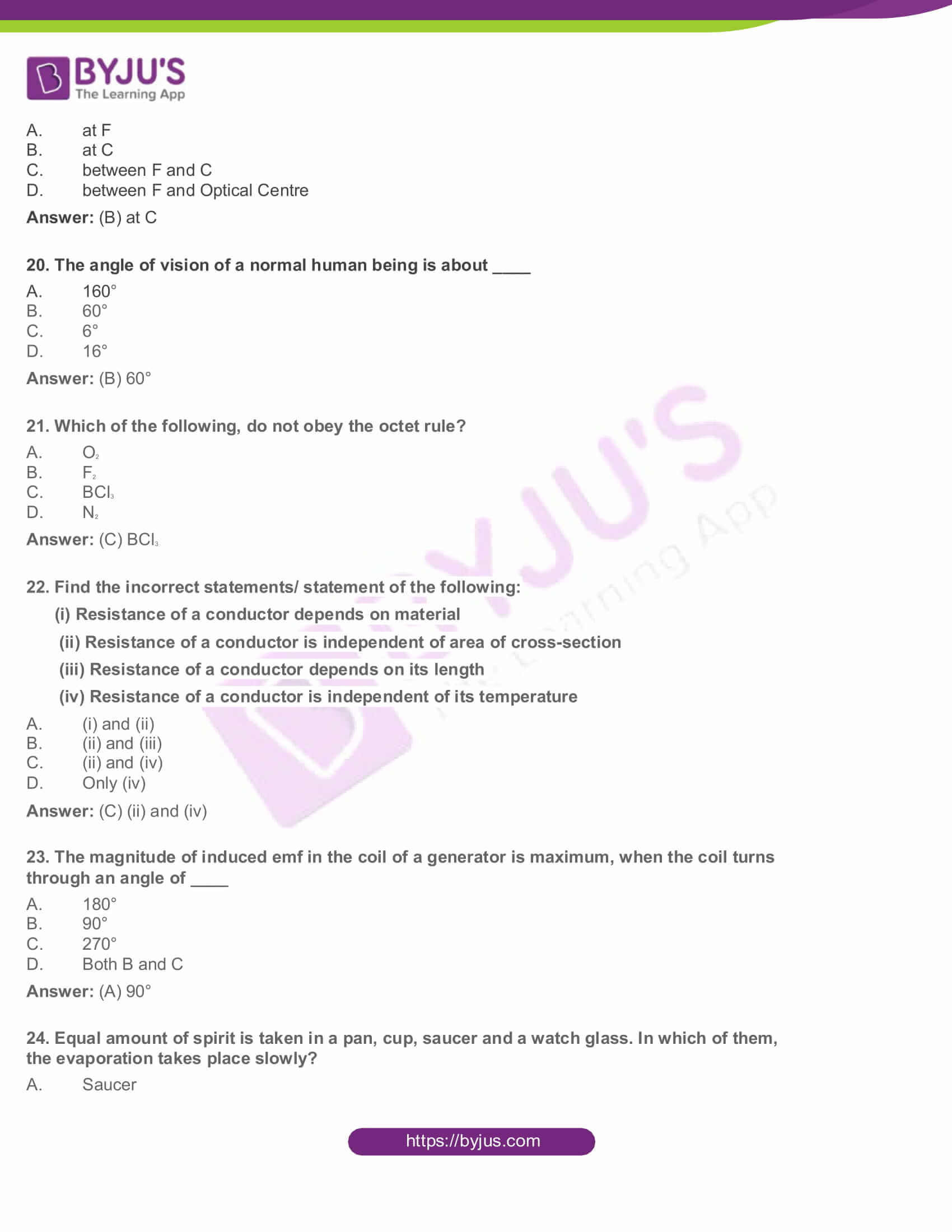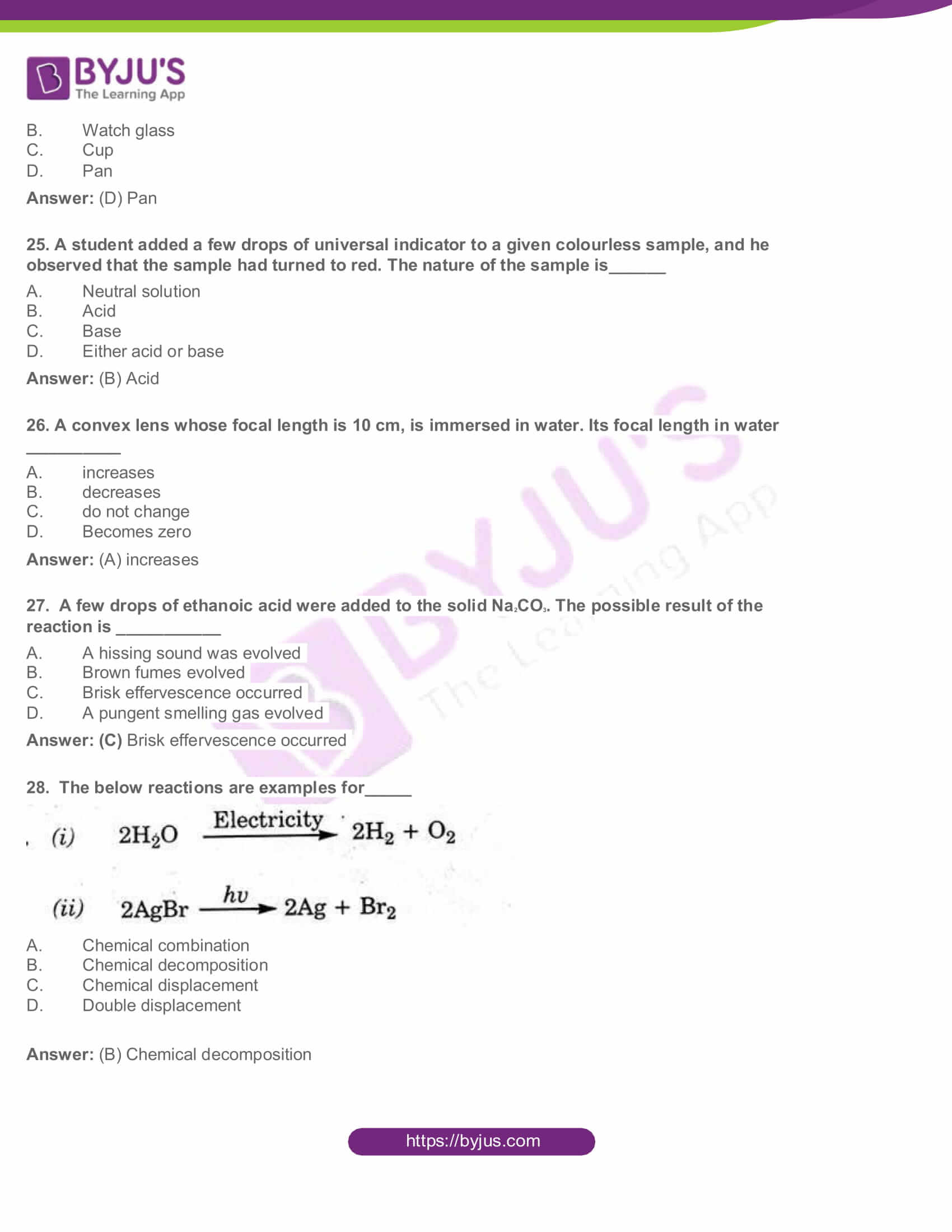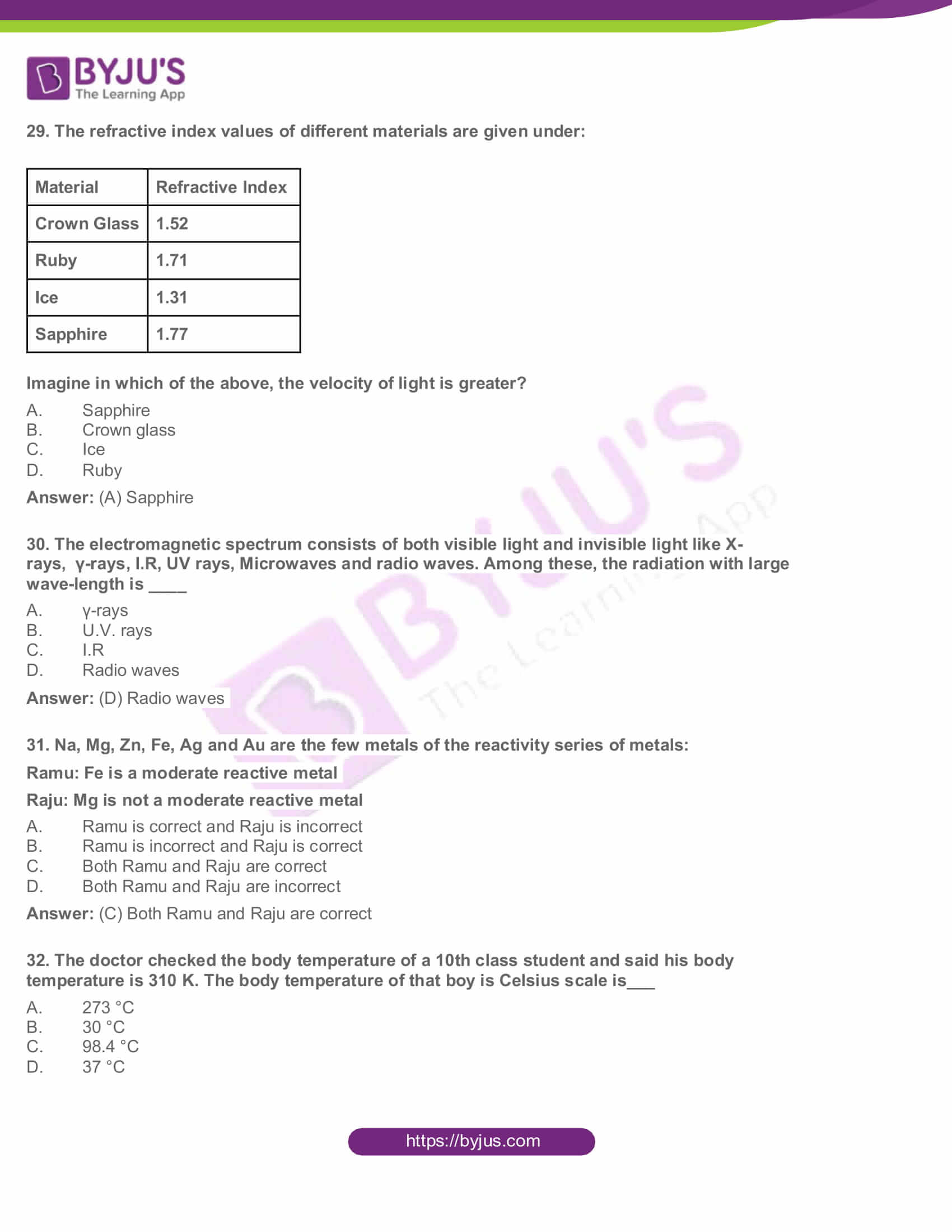Physical Science

SECTION A

Section-I 4 x 1=4

1. We use P.V.C pipes for water supply instead of metal pipes. Why?

Answer: P.V.C pipes are lighter and also cost lesser than metal pipes. Also, PVC pipes do not rust or corrode like the metal pipes.

2. Optical Fibre Cable (OFC) is often used in telecommunications. What is the working principle behind the OFC?

Answer: Total internal reflection is the basic principle behind working of optical fibre. An optical fibre is a very thin fibre made of glass (or) plastic having radius about a micrometer (10-6 m). A

bunch of such thin fibres forms a light pipe.

3.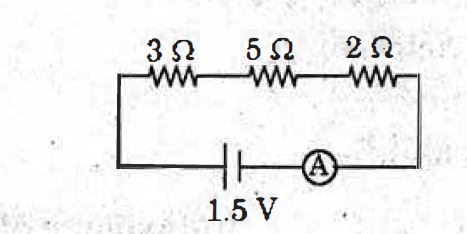Find the quantity of current in the above circuit.

Answer: Series connection resistance= 3+5+2 = 10 ohm

Acc ohms law, V=IR,

Quantity of current (I) = V/R

I = 1.5/10= 0.15 A

4. Roasting and Calcination are the methods used to extract Crude·metals from ores. What is the difference between Roasting and Calcination?

Answer: Roasting is a pyrochemical process in which the ore is heated in the presence of oxygen or air below its melting point. The products (like metal oxide from sulphide ore) obtained in the process also are in solid state. Meanwhile, Calcination is a process of heating the ore strongly in the absence of air or oxygen. During calcination, carbonate is converted to its oxide.

Section- II 5 x 2=10

5. Balance the chemical equation by indicating the physical state of the substances for the following reaction:

Barium chloride and Sodium sulphate aqueous solutions react to give Barium sulphate precipitate and aqueous Sodium chloride.

Answer: Sodium sulphate reacts with barium chloride to give white precipitate, barium sulphate.

Na2SO4 + BaCl2→ BaSO4 + NaCl

This equation is almost balanced. Except for Na and Cl, rest is all equal, so we just have to add 2 before NaCl.

Hence, Na2SO4 + BaCl2→ BaSO4 + 2NaCl

6. A convex lens is made of five different materials as shown in the figure. How many images does it form ? Why ? .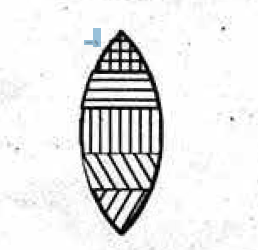Answer: If a convex lens is made of five different materials then the lens will have five different refractive indices or different focal lengths. So, the lens will form five different images, one image each for every refractive.

7. The electronic configuration of Sodium is. 1s22s22p63s1. What is the information that it gives?

Answer: Sodium is a vital element of the periodic table. The atomic number of Sodium is 11 and the total number of electrons is also 11 in the Sodium atom. To learn more about the electronic configuration of Sodium, check out.

8. We can save the household wiring and devices by using fuses. Write any four points appreciating the role of fuse.

Answer: To prevent damages due to overloading we can connect an electric fuse to the household circuit in such a way that the entire current from the mains is passed through the fuse. When the current in the fuse exceeds 20A, the wire will heat up and melt. The circuit then opens up and prevents the flow of current into the household circuit. So, all the electric devices are saved from any damage caused by overload. Thus, we can save the household wiring and devices by using fuses.

9. Potassium, Sodium, Magnesium are high reactive metals and occur as chlorides in nature. Suggest and explain the suitable method for the extraction of the above metals from their ores.

Answer: Metals that are highly reactive such as Potassium, Sodium, Magnesium are extracted from their ores using electrolytic reduction method. During electrolytic reduction, the metals that are positively charged are deposited at the Cathode (the negatively charged electrode).

Section-III 4 x 4=16

10. Sudheer wants to verify the laws of reflection. What apparatus does he require to prove them? State the laws of reflection and write the experimentation process he follows.

Answer: Law of reflection is defined as:

1. The law of reflection defines that upon reflection from a smooth surface, the angle of the reflected ray is equal to the angle of the incident ray, with respect to the normal to the surface that is to a line perpendicular to the surface at the point of contact.
2. The reflected ray is always in the plane defined by the incident ray and the normal to the surface at the point of contact of the incident ray.

Now, below we have given the steps followed by Sudeesh in the experiment to verify the laws of reflection.

Aim: Verification of laws of reflection

Required material: mirror strip, drawing board, white paper, pins,clamps, scale and pencil

Procedure: Take a drawing board and fix a white paper on it with the help of clamps. Draw a straight line AB at the centre of the paper and also a normal (ON) to AB at the point ‘O’. Draw a straight line PQ making certain angle (angle i) with ON as shown in figure below. Fix two pins at the points P and Q on the paper vertically. Observe the image PI of the pin P and QI of the pin Q, in the mirror kept along the line AB. Fix two more pins R and S such that they are in the same line as that of PI and QI. Join R, S and O as in the figure below. Measure the angle between RS and ON (angle of reflection). You will find that angle of incidence = angle of reflection.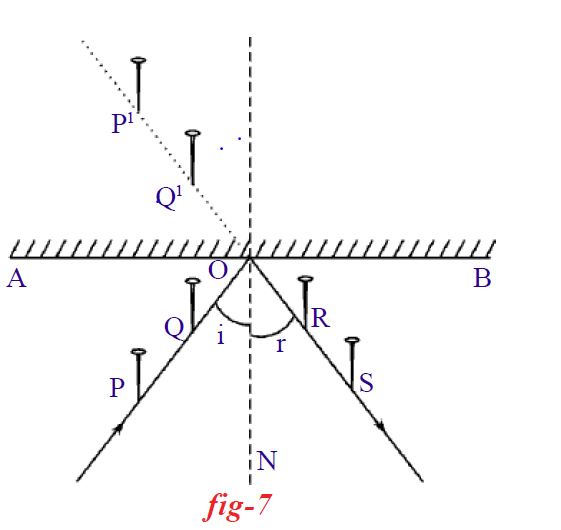Repeat for two more incident angles (say 45 degree and 60 degree). Now, see the head of the pins9 (Same as draw a line at right angles to the edge of the edge of the mirror to act as the normal- ON.). Raise one pin and press another pin. Now see an image of two pins from the other side of the normal and place a pin vertically so that it is in line with images of the other two pins. Similarly, place one more pin at some distance from this pin such that images of the pin-head are aligned. Visualise the plane containing incident ray, reflected ray and normal. You may place a paper on this plane. Raise another pin and visualise again.

Or

10. How can you verify with experiment the statement “The magnetic field lines are closed loops”.

Answer: The magnetic field lines are closed loops because a magnet is always a dipole and as a result, the net magnetic flux of a magnet is always zero. Now, learn more about Faraday’s laws and the experiment to support it from here.

11. Elements of one short period of the Periodic Table are given below in the order from left to right.

Li, Be, B, C, N, F, Ne

(i) To which period do these elements belong?

(ii) One element of this period is missing. Which is the missing element and where should it be placed?

(iii) Which of the above elements belong to the family of halogens? What is its electronegativity value?

(iv) How does the metallic character vary in the Period?

Answer: (i) These elements belong to the second (2nd) period.

(ii) The missing element from this period is O and it is placed between N and F.

(iii) F (Fluorine) belong to the family of halogens. This period is arranged in the increasing order of electron affinity. Fluorine also has the highest electronegativity in the series at 4.

(iv) Metals generally show less electronegative character. In compounds, they generally show a tendency to remain as positive ions. This property is often termed as electropositive character. Metals are electropositive elements. Normally, we find metals on the left side and non-metals on the right side of the periodic table. This means metallic character decreases while non-metallic character increases as we move along a period (from left to right).

Or

11. The arrangement of electrons in different shells of atoms of the 18th group elements is given in the table.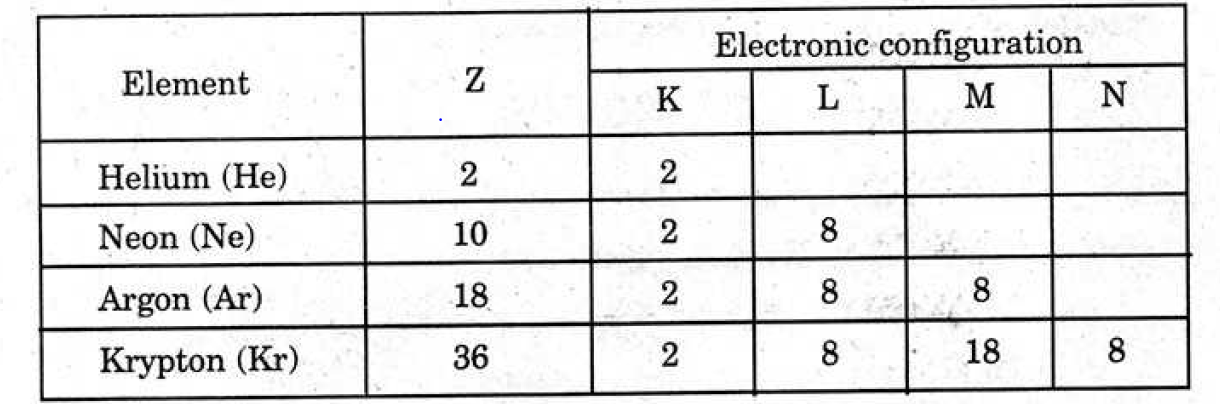(i) What is the general electronic configuration of the above elements, except for He?

(ii) What is the valency of Argon?

(iii) Write Luis dot structure of Neon.

(iii) Why do the above elements not take part in bond formation?

Answer: (i) The general electronic configuration of the above elements are like Neon(Ne)- (2,8), Argon(Ar)- (2,8,18) and Krypton(Kr)-(2,8,18,8)

(ii) The elements in the 18th group are noble gases expected to be inert and Argon is one among them. Hence, the valency of Argon is expected to be zero.

(iii) The Lewis dot structure of the atom of noble gas Neon is given below: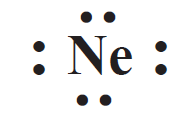(iv) The noble gases (except for helium, which has a duplet of electrons) have a particularly stable outer shell configuration of eight (octet) electrons, ns2np6.and so are considered to be inert; they do not readily take part in chemical reactions.

12. What are the factors that affect the process of evaporation? Explain with suitable examples.

Answer: Expose liquid to air and the molecules at the surface keep on escaping from the surface till the entire liquid disappears into air. This process is called evaporation. During this process, the energy of the molecules inside the liquid decreases, and they slow down. They transfer this energy to escaping molecules during the collisions.“The process of escaping of molecules from the surface of a liquid at any temperature is called evaporation.” Rate of evaporation of a liquid depends on its surface area, temperature and amount of vapour already present in the surrounding air. To support this, see the example given below:

Take a few drops of spirit (say 1 ml) in two petri dishes (a shallow glass or plastic cylindrical lidded dish used in the laboratory) separately. Keep one of the dishes containing spirit under a ceiling fan and switch on the fan. Keep another dish with its lid closed. Observe the quantity of spirit in both dishes after 5 minutes. The spirit in the dish that is kept under the ceiling fan disappears, whereas you will find some spirit left in the dish that is kept in the lidded dish. Now, determine the reason for faster evaporation of spirit kept under the fan. If air is blown over the liquid surface in an open pan or petri dish, the number of molecules returned is reduced to a large extent. This is because any molecule escaping from the surface is blown away from the vicinity of the liquid. This increases the rate of evaporation. This is the reason why the spirit in petri dish, that is kept under the ceiling fan evaporates quickly when compared to that kept closed. You will notice that clothes dry faster when a wind is blowing.

Or

12. Alkanes are considered as Paraffin. So, they undergo substitution reactions but not addition reactions. Explain with suitable examples.

Answer: Substitution reaction is the reaction in which an atom or a group of atoms in a given compound is replaced by another atom or group of atoms. Alkanes, the saturated hydrocarbons are chemically least reactive. Therefore, they are also called paraffins. However, they undergo some chemical changes under suitable conditions, which are substitution reactions.

For example, methane (CH4) reacts with chlorine in the presence of sunlight. Hydrogen atoms of CH4 are replaced by chlorine atoms.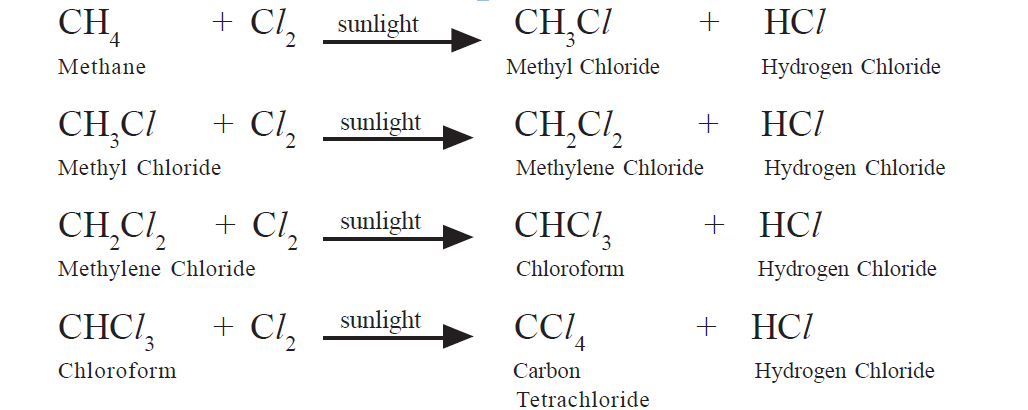13. Revathi is a front bench student. She is unable to draw the picture drawn on the blackboard. She got permission from the teacher and sat in the back row. What could be the defect that Revathi is suffering from? Draw the diagram, which shows the correction of the above defect.

Answer: Revathi is suffering from Hypermetropia, also known as “far-sightedness”. A person with hypermetropia can see distant objects clearly, but cannot see objects at near distances, because the minimum focal length of eye lens for the person of hypermetropia is greater than 2.27 cm. In such cases, the rays coming from a nearby object, after refraction at the eye lens, forms an image beyond the retina. The point of minimum distance at which the eye lens can form an image on the retina is called near point (d). The people with defect of hypermetropia cannot see objects placed between near point (H) and point of the least distance of distinct vision (L). Eye lens can form a clear image on the retina when any object is placed beyond near point. To correct the defect of hypermetropia, we need to use a lens, which forms an image of an object beyond near point, when the object is between near point (H) and least distance of distinct vision (L). This is possible only when a double convex lens is used. From the diagram below, see correction of the defect: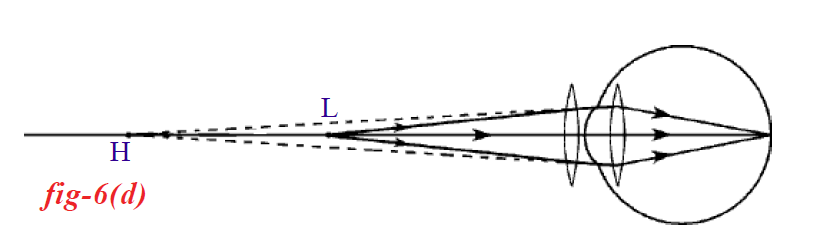Or

13. Draw a neat diagram showing that a base solution in water conducts electricity. Why is it then that the solution of sugar/ glucose in water do not conduct electricity?

Answer: Sugar or glucose in water do not conduct electricity as the sugar (C12H22O11) dissolves in water to produce sugar molecules, which are usually neutral (not charged). So these are unable to move to the opposite ends of the electrodes like the ions.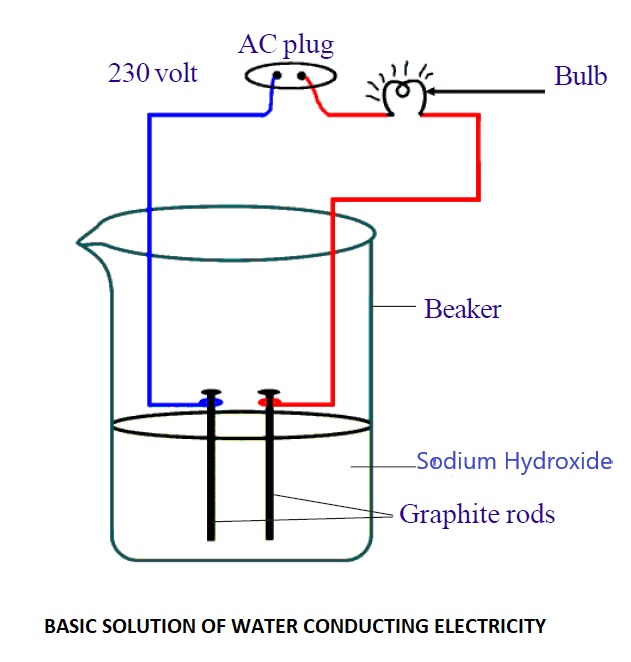Part B

Section- IV 20 x ½ = 10

14. BaCl2+ Na2SO4 → BaSO4 + 2NaCl represents_____

(i) Decomposition reaction

(ii) Displacement reaction

(iii) Precipitation reaction

(iv) Double displacement reaction

1. Only i
2. (ii) and (iii)
3. (iii) and (iv)
4. Only (iv)

15. A test tube immersed at a certain angle in a tumbler of water to have a mirror surface for a certain viewing position. It is due to _____

1. Reflection
2. Refraction
3. Total internal reflection
4. Scattering

16. In torches, search lights and headlights of vehicles, the bulb is placed____

1. between the pole and the focus of the reflector
2. at the focus of the reflector
3. at the centre of curvature of the reflector
4. between the focus and the centre of curvature of the reflector

Answer: (B) at the focus of the reflector

17. The unit of refractive index is _____

1. Centimetre
2. Diapter
3. Degree
4. No Units

18. Match the following:

 (i) Scattering P. Eye defect (ii) Dispersion Q. VIBGYOR (iii) Power of Lens R. Retina (iv) Cones and Rods S. Colour of the Sky
1. (i)- (S) (ii)- Q (iii)-R (iv)-P
2. (i)-Q (ii)-S (iii)-P (iv)-R
3. (i)- S (ii)-Q (iii)-P (iv)-R
4. (i)-Q (ii)-S (iii)-R (iv)-P

Answer: (C) (i)- S (ii)-Q (iii)-P (iv)-R

19. A real and inverted image of the same size is formed by a convex lens, when the object is placed__________

1. at F
2. at C
3. between F and C
4. between F and Optical Centre

20. The angle of vision of a normal human being is about ____

1. 160°
2. 60°
3. 16°

21. Which of the following, do not obey the octet rule?

1. O2
2. F2
3. BCl3
4. N2

22. Find the incorrect statements/ statement of the following:

(i) Resistance of a conductor depends on material

(ii) Resistance of a conductor is independent of area of cross-section

(iii) Resistance of a conductor depends on its length

(iv) Resistance of a conductor is independent of its temperature

1. (i) and (ii)
2. (ii) and (iii)
3. (ii) and (iv)
4. Only (iv)

23. The magnitude of induced emf in the coil of a generator is maximum, when the coil turns through an angle of ____

1. 180°
2. 90°
3. 270°
4. Both B and C

24. Equal amount of spirit is taken in a pan, cup, saucer and a watch glass. In which of them, the evaporation takes place slowly?

1. Saucer
2. Watch glass
3. Cup
4. Pan

25. A student added a few drops of universal indicator to a given colourless sample, and he observed that the sample had turned to red. The nature of the sample is______

1. Neutral solution
2. Acid
3. Base
4. Either acid or base

26. A convex lens whose focal length is 10 cm, is immersed in water. Its focal length in water __________

1. increases
2. decreases
3. do not change
4. Becomes zero

27. A few drops of ethanoic acid were added to the solid Na2CO3. The possible result of the reaction is ___________

1. A hissing sound was evolved
2. Brown fumes evolved
3. Brisk effervescence occurred
4. A pungent smelling gas evolved

28. The below reactions are examples for_____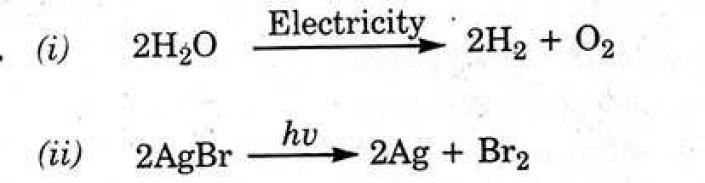1. Chemical combination
2. Chemical decomposition
3. Chemical displacement
4. Double displacement

29. The refractive index values of different materials are given under:

 Material Refractive Index Crown Glass 1.52 Ruby 1.71 Ice 1.31 Sapphire 1.77

Imagine in which of the above, the velocity of light is greater?

1. Sapphire
2. Crown glass
3. Ice
4. Ruby

30. The electromagnetic spectrum consists of both visible light and invisible light like X-rays, γ-rays, I.R, UV rays, Microwaves and radio waves. Among these, the radiation with large wave-length is ____

1. γ-rays
2. U.V. rays
3. I.R

31. Na, Mg, Zn, Fe, Ag and Au are the few metals of the reactivity series of metals:

Ramu: Fe is a moderate reactive metal

Raju: Mg is not a moderate reactive metal

1. Ramu is correct and Raju is incorrect
2. Ramu is incorrect and Raju is correct
3. Both Ramu and Raju are correct
4. Both Ramu and Raju are incorrect

Answer: (C) Both Ramu and Raju are correct

32. The doctor checked the body temperature of a 10th class student and said his body temperature is 310 K. The body temperature of that boy is Celsius scale is___

1. 273 °C
2. 30 °C
3. 98.4 °C
4. 37 °C